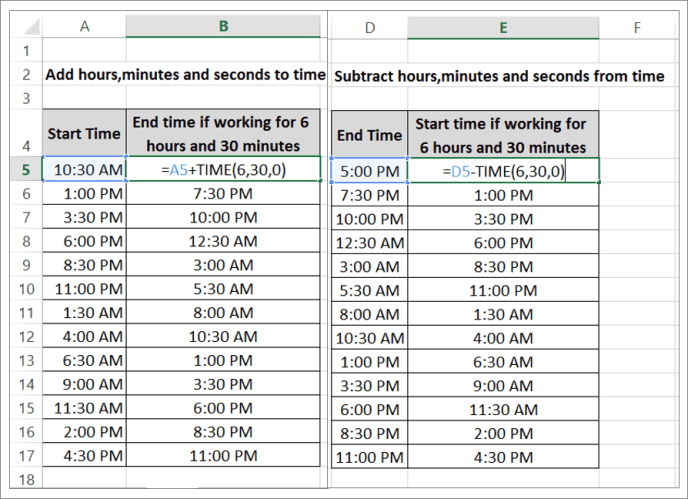[email protected] +1 203-349-9909 / +91-8080042523

# Calculate Elapsed Time in Excel

We want to calculate elapsed time in excel i.e. Add or Subtract hours, minutes and seconds to the time.

## How to calculate elapsed time in Excel?

Use the following formula TIME (HOURS, MINUTES, SECONDS) to calculate elapsed time in excel. After entering the formula, you may require changing the cell format as per your requirement. In the elapsed time example below, We have two cases:

In the First case, you have to add 6 hours and 30 minutes to all the working hours/times on the list.

Also, Watch out Free Excel Training Lessons which has helped 20,000+ users in learning basic excel tips & tricks. Click here

In the Second case, the End time of a person is fixed so you have to subtract 6 hours and 30 minutes to all the working hours/times in the list.(Time Calculator Function in Excel)

To add a certain number of hours to an existing time value, simply use the TIME function to build a new time value and then add them together. The following Time Calculator formula adds 30 minutes to the existing time, resulting in a time value of 3:00 p.m =”2:30:00 PM” + TIME (0, 30, 0).